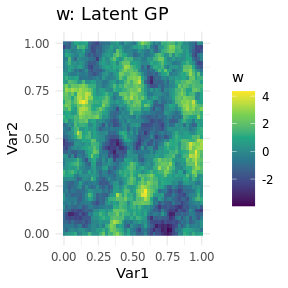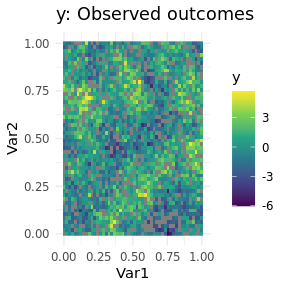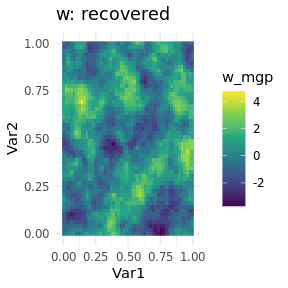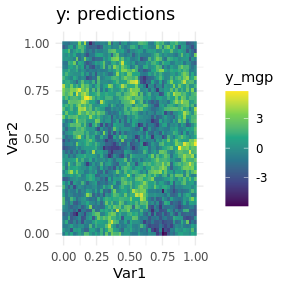# MGPs for univariate spatial gridded data

#### 2021-06-21

This is the simplest case for Meshed GPs. We start by generating some data

library(magrittr)
library(dplyr)
library(ggplot2)
library(meshed)
set.seed(2021)

SS <- 50 # coord values for jth dimension
dd <- 2 # spatial dimension
n <- SS^2 # number of locations
p <- 3 # number of covariates

xlocs <- seq(0, 1, length.out=SS)
coords <- expand.grid(list(xlocs, xlocs))

sigmasq <- 1.5
nu <- 0.5
phi <- 10
tausq <- .1

# covariance at coordinates
CC <- sigmasq * exp(-phi * as.matrix(dist(coords)))
# cholesky of covariance matrix
LC <- t(chol(CC))
# spatial latent effects are a GP
w <- LC %*% rnorm(n)
# measurement errors
eps <- tausq^.5 * rnorm(n)

# covariates and coefficients
X <- matrix(rnorm(n*p), ncol=p)
Beta <- matrix(rnorm(p), ncol=1)

# univariate outcome, fully observed
y_full <- X %*% Beta + w + eps

# now introduce some NA values in the outcomes
y <- y_full %>% matrix(ncol=1)
y[sample(1:n, n/5, replace=FALSE), ] <- NA

simdata <- coords %>%
cbind(data.frame(Outcome_full= y_full,
Outcome_obs = y,
w = w))

simdata %>% ggplot(aes(Var1, Var2, fill=w)) +
geom_raster() +
scale_fill_viridis_c() +
theme_minimal() + ggtitle("w: Latent GP")simdata %>% ggplot(aes(Var1, Var2, fill=y)) +
geom_raster() +
scale_fill_viridis_c() +
theme_minimal() + ggtitle("y: Observed outcomes")We now fit the following spatial regression model $y = X \beta + w + \epsilon,$ where $$w \sim MGP$$ are spatial random effects, and $$\epsilon \sim N(0, \tau^2)$$. For spatial data, an exponential covariance function is used by default: $$C(h) = \sigma^2 \exp( -\phi h )$$ where $$h$$ is the spatial distance.

The prior for the spatial decay $$\phi$$ is $$U[l,u]$$ and the values of $$l$$ and $$u$$ must be specified. We choose $$l=1$$, $$u=30$$ for this dataset.1

Setting verbose tells spmeshed how many intermediate messages to output while running MCMC. We set up MCMC to run for 3000 iterations, of which we discard the first third. We use the argument block_size=25 to specify the coarseness of domain partitioning. In this case, the same result can be achieved by setting axis_partition=c(10, 10).

Finally, we note that NA values for the outcome are OK since the full dataset is on a grid. spmeshed will figure this out and use settings optimized for partly observed lattices, and automatically predict the outcomes at missing locations. On the other hand, X values are assumed to not be missing.

mcmc_keep <- 1000
mcmc_burn <- 1000
mcmc_thin <- 2

mesh_total_time <- system.time({
meshout <- spmeshed(y, X, coords,
#axis_partition=c(10,10), #same as block_size=25
block_size = 25,
n_samples = mcmc_keep, n_burn = mcmc_burn, n_thin = mcmc_thin,
verbose = 0,
prior=list(phi=c(1,30))
)})

We can now do some postprocessing of the results. We extract posterior marginal summaries for $$\sigma^2$$, $$\phi$$, $$\tau^2$$, and $$\beta_2$$. The model that spmeshed targets is a slight reparametrization of the above:2 $y = X \beta + \lambda w + \epsilon,$ where $$w\sim MGP$$ has unitary variance. This model is equivalent to the previous one and in fact we find $$\sigma^2=\lambda^2$$.

summary(meshout$lambda_mcmc[1,1,]^2) #> Min. 1st Qu. Median Mean 3rd Qu. Max. #> 1.199 1.777 1.963 2.042 2.232 4.635 summary(meshout$theta_mcmc[1,1,])
#>    Min. 1st Qu.  Median    Mean 3rd Qu.    Max.
#>   3.043   6.555   7.463   7.514   8.464  13.989
summary(meshout$tausq_mcmc[1,]) #> Min. 1st Qu. Median Mean 3rd Qu. Max. #> 0.06073 0.10772 0.12262 0.12063 0.13441 0.18332 summary(meshout$beta_mcmc[1,1,])
#>    Min. 1st Qu.  Median    Mean 3rd Qu.    Max.
#> -0.8480 -0.8017 -0.7930 -0.7926 -0.7833 -0.7474

We proceed to plot predictions across the domain along with the recovered latent effects.

# process means
wmesh <- data.frame(w_mgp = meshout$w_mcmc %>% summary_list_mean()) # predictions ymesh <- data.frame(y_mgp = meshout$yhat_mcmc %>% summary_list_mean())

outdf <- meshout$coordsdata %>% cbind(wmesh, ymesh) %>% left_join(simdata) outdf %>% ggplot(aes(Var1, Var2, fill=w_mgp)) + geom_raster() + scale_fill_viridis_c() + theme_minimal() + ggtitle("w: recovered")outdf %>% ggplot(aes(Var1, Var2, fill=y_mgp)) + geom_raster() + scale_fill_viridis_c() + theme_minimal() + ggtitle("y: predictions")1. spmeshed implements a model which can be interpreted as assigning $$\sigma^2$$ a folded-t via parameter expansion if settings$ps=TRUE, or an inverse Gamma with parameters $$a=2$$ and $$b=1$$ if settings\$ps=FALSE, which cannot be changed at this time. $$\tau^2$$ is assigned an exponential prior.↩︎

2. At its core, spmeshed implements the spatial factor model $$Y(s) = X(s)\beta + \Lambda v(s) + \epsilon(s)$$ where $$w(s) = \Lambda v(s)$$ is modeled via linear coregionalization.↩︎